## ICSE Physics Previous Year Question Paper 2008 Solved for Class 10

ICSE Paper 2008
PHYSICS

(Two hours)
Answers to this Paper must he written on the paper provided separately.
You will not be allowed to write during the first 15 minutes.
This time is to he spent in reading the question paper.
The time given at the head of this Paper is the time allowed for writing the answers.
Section I is compulsory. Attempt any four questions from Section II.
The intended marks for questions or parts of questions are given in brackets [ ].

SECTION-I (40 Marks)

Attempt all questions from this Section.

Question 1:
(a) Name the SI unit of:
(ii) Linear momentum
(ii) Rate of change of momentum.  

(b) A body of mass 1.50 kg is dropped from the 2nd floor of a building which is at a height of 12 m. What is the force acting on it during its fall ?
(g = 9.80 ms-2)  

(c) When an arrow is shot from a bow, it has kinetic energy in it. Explain briefly from where does it get its kinetic energy ?  

(d) What energy conversions take place in the following when they are working:
(i) Electric toaster (ii) Microphone ?  

(e) Copy the diagram of the forearm given below, indicate the positions of Load, Effort and Fulcrum.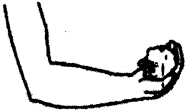(a) (i) Kg ms-1
(ii) Kg ms-2 or N (Newton)

(b) We know that  F = mg
= 1.5 × 9.8 = 14.7 N

(e) When bow is stretched, work is done on it which has elastic potential energy and on releasing it, potential energy converts into kinetic energy.

(d) (i) Electric energy to heat energy.
(ii) Sound energy to electrical energy

(e)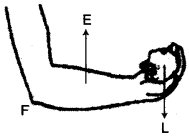Question 2:
(a) (i) A monochromatic beam of light of wavelength X passes from air into a glass block. Write an expression to show the relation between the speed of light in air and the speed of light in glass.
(ii) As the ray of light passes from air to glass, state how the wavelength of light changes. Does it increase, decrease or remain constant ?  

(b) In what way will the temperature of water at the bottom of a water fall be different from the temperature at the fop ? Give a reason for your answer.  

(c) A radar sends a signal to an aeroplane at a distance 45 km away with a speed of 3 x 108 ms-1. After how long is the signal received back from the aeroplane ?  

(d) Draw a ray diagram to illustrate the determination of the focal length of a convex lens using an auxiliary plane mirror.  

(e) What is meant by primary colours ? Name the primary colours of light.  

(a) (i)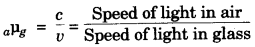(ii)Refractive index (μ) increases with decrease in wavelength (λ).
∵ Wavelength of light decreases.

(b) Temperature at bottom is more, because the potential energy at top converts to heat energy when water reaches at bottom, as a result temperature of water at the bottom increases.

(c)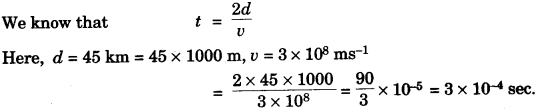(d)(e) Answer has not given due to out of present syllabus.

Question 3:
(a) (i) Sketch a graph to show the change in potential difference across the ends of an ohmic resistor and the current flowing in it. Label the axes of your graph. (ii) What does the slope of the graph represent ? 

(b) Draw a labelled diagram of the staircase wiring for a dual control switch showing a bulb in the circuit.  

(c) The electrical gadgets used in a house such as bulbs, fans, heater, etc., are always connected in parallel, NOT in series. Give two reason for connecting them in parallel.  

(d) An electrical heater is rated 4 kW, 220 V. Find the cost of using this heater for 12 hours if one kWh of electrical energy costs Rs. 3.25.  

(e) State one point of similarity and one point of difference between an a.c. generator and a d.c. motor. 

(a) (i)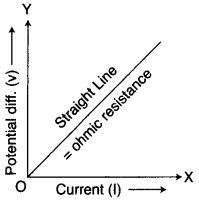(ii) Slope = $$\frac { \Delta V }{ \Delta I }$$ = R (Resistance)

(b)Either of switch is ON, Bulb will be ON.

(c) (i) In parallel, the voltage across or potential across is same.
(ii) If one of the appliance is off or not working atleast others will work.

(d) Given : P = 4 kW, V = 220 V, t = 12 hrs
E = P × t = 4 × 12 = 48 kWh
Cost = 48 × 3.25 = Rs. 156.

(e) Difference: AC generator converts mechanical energy to electrical while DC motor converts electrical energy to mechanical energy.
Similarity: In both, the coil or armature moves or rotate in uniform magnetic field.

Question 4:
(a) Two isosceles right-angled glass prisms are placed near each other as shown in the figure.Complete the path of the light ray entering the first isosceles right-angled glass prism till it emerges from the second identical prism.  

(b) A certain quantity of ice at 0°C is heated till it changes into steam at 100°C. Draw a time-temperature heating curve to represent it. Label the two phase changes in your graph.  

(c) What is meant by work function of a metal ?  

(d) What is radioactivity ?  

(e) Mention any two differences between nuclear energy and chemical energy.  

(a)(b)Two phase changes in graph are :
BC —-> Ice to water
DE —-> Water to Vapour

(c) Work function: Work function is the maximum amount of energy required eject electron from metal surface. It is measured in eV.

(d) Radioactivity : Radioactivity is nuclear phenomenon. It is the spontaneous emission of α, β and γ radiation from nuclei of an atom.

(e) Answer has not given due to out of present syllabus.

SECTION-II (40 Marks)

Attempt any four questions from this Section.

Question 5:
(a) (i) A stone of mass 64.0 g is thrown vertically upward from the ground with an initial speed of 20.0 ms-1. The gravitational potential energy at the ground level is considered to he zero. Apply the principle of conservation of energy and calculate the potential energy at the maximum height attained by the
stone, (g = 10 ms-2).  

(ii) Using the same principle, state what will be the total energy of the body at its half-way point ?  

(b) Define ‘joule’, the SI unit of work and establish a relationship between the SI and CGS unit of work. 

(c) (i) Draw a labelled diagram of a block and tackle system of pulleys with two pulleys in each block. Indicate the directions of the load, effort and tension in the string.
(ii) Write down the relation between the load and the effort of the pulley system.  

(a) (i)(ii) Total energy of the stone would remain same i.e., 12.8 J.

(b) Joule: One Joule is the work done when a force of 1 N acts on a body and it displaces by 1 m in its own direction.
The SI unit of work is Joule.
C.G.S. unit of work = ergs
1 J = 1 N x 1 m
= 106 dyne x 100 cm
= 107 dyne/cm
∴  1 J = 107 ergs

(c) (i) Block and tackle system of a pulley.(ii)Question 6:
(a) What is meant by the ‘aperture’ and ‘shutter speed’ of a camera ? How are they related ?  

(b) (i) Draw a labelled ray diagram to illustrate (1) critical angle (2) total internal reflection, for a ray of light moving from one medium to another.
(ii) Write a formula to express the relationship between refractive index of the denser medium with respect to rarer medium and its critical angle for that pair of media.  

(c) (i) The diagram given alongside shows a ray of light incident on an equilateral glass prism placed in minimum deviation position. Copy the diagram and complete it to show the path of the refracted ray and the emergent ray.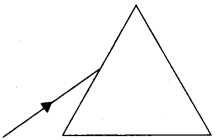(ii) How are angle of incidence and angle of emergence related to each other in this position of the prism ?  

(a) Answer has not given due to out of present syllabus.

(b) (i)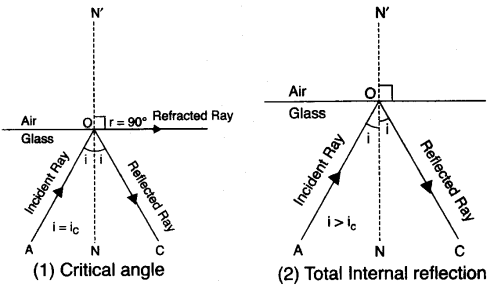(ii) Refractive index of air with respect to glass is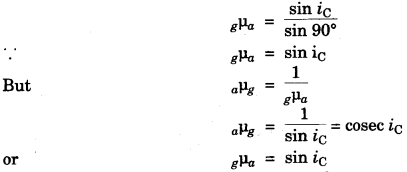(c) (i) For minimum deviation : i = e, r1 = r2, δ = δm(ii) Angle of incidence (i) = Angle of emergent (e)

Question 7:
(a) (i) What is meant by an echo ? Mention one important condition that is necessary for an echo to be heard distinctly.
(ii) Mention one important use of echo.  

(b) (i) What is the name given to a cylindrical coil whose diameter is less in comparison to its length ?
(ii) If a piece of soft iron is placed inside the current carrying coil, what is the name given to the device ?
(iii) Give one use of the device named by you in (b) (ii) above.  

(c) (i) Sometimes when a vehicle is driven at a particular speed, a rattling sound is heard. Explain briefly, why this happens and give the name of the phenomenon taking place.
(ii) Suggest one way by which the rattling sound could be stopped.  

(a) (i) Echo: It is reflection of sound when it strikes the rigid surface. To hear an echo distinctly, the minimum distance between the reflecting surface and the listener must be approx. 17 m.
(ii) Use of echo in SONAR to find the depth of an obstacle in sea.

(b) (i) Solenoid
(ii) In Electromagnet.
(iii) In Electric bell (d.c.)

(c) (i) Phenomenon is resonance. When the frequency of engine becames equal to the body of vehicle, some parts of vehicle start vibrating violently. Due to resonance rattling sound is heard.
(ii) Rattling sound can be stopped by changing the speed of vehicle.

Question 8:
(a) (i) Why does a magnetic needle show a deflection when brought close to a current carrying conductor ?
(ii) A wire bent into a circle carries current in an anti clockwise direction. What polarity does this face of the coil exhibit ?

(b) Three resistors of 6.0 Ω, 2.0 Ω and 4.0 Ω respectively are joined together as shown in the figure.The resistors are connected to an ammeter and to a cell of emf 6.0 V. Calculate :
(i) The effective resistance of the circuit.
(ii) The current drawn from the cell. 

(c) (i) How does the heat produced in a wire or a conductor depend upon the :
(1) current passing through the conductor.
(2) resistance of the conductor ?
(ii) Draw a simple sketch of a step down transformer. Label the different parts in the diagram.

(a) (i) It is because the current carrying coil produces magnetic field around it.
(ii) North pole is exhibited.(b) (i) Resistors R2 and R3 are in series.
∴ Equivalent resistance R = 2 + 4 = 6Ω and this is parallel to R1 = 6Ω.(ii) Let V = IR
$$I=\frac { V }{ R } =\frac { 6 }{ 3 } =2A$$

(c) (i) (1) Heat produced in a wire is directly proportional to the square of current through the conductor (H ∝ I2)
(2) Heat produced in a wire is directly proportional to the resistance of conductor(H ∝ R)
(ii)Question 9:
(a) (i) Define heat capacity of a given body. What is its SI unit ?
(ii) What is the relation between heat capacity and specific heat capacity of a substance ?  

(b) A linear object is placed on the axis of a lens. An image is formed by refraction in the lens. For all positions of the object on the axis of the lens, the positions of the image are always between the lens and the object.
(i) Name the lens.
(ii) Draw a ray diagram to show the formation of the image of an object placed in front of the lens at any position of your choice except infinity.  

(c) A piece of ice of mass 40 g is dropped into 200 g of water at 50°C.Calculate the final temperature of water after all the ice has melted.
(specific heat capacity of water = 4200 J kg-1 °C,
specific latent heat of fusion of ice = 336 x 103 J kg-1)  

(a) (i) Heat Capacity: Heat capacity is the amount of heat required to raise the temperature of a body by 1°C. Its SI unit is J/°C.
(ii) Heat capacity = mass x specific heat capacity.

(b) (i) Concave lens.
(ii)(c)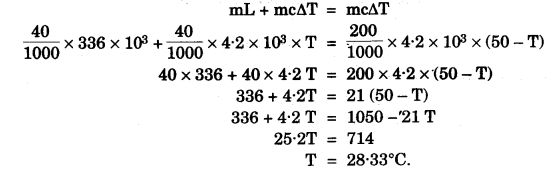Question 10:
(a) (i) When does the nucleus of an atom become radioactive ?
(ii) How is the radioactivity of an element affected when it undergoes a chemical change to form a chemical compound ?
(iii) Name the product of nuclear fission which is utilized to bring about further fission of $$_{ 92 }^{ 235 }{ U }$$.  

(b) (i) Mention one use and one harmful effect of radioactivity.
(ii) Give one source of background radiation.  

(c) (i)The above diagram shows an electron gun of a hot cathode ray tube,
1. Name the parts X and Y.
2. A 6V d.c. source and a 1000V d.c. source are available. Show how these sources should be connected to the terminals of X and Y so as to obtain a focused beam of fast moving electrons.
(ii) Give one use of a cathode ray tube.